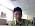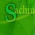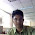Monday, 2 July, 2007

My favourite C questions

1. Write a "Hello World" program in 'C' without using a semicolon.

void main()
{
if(printf("Hello World\n"))
{
}
}

2. Write a C++ program without using any loop (if, for, while etc) to print numbers from 1 to 100 and 100 to 1;

I haven't tried this code, but i think that it will work, please check and tell me...

#include <stdio.h>

int one_round = 0;

int loop(int a){
(one_round == 0)?printf("%d\n", a++):printf("%d\n", a--);
(a == 100)? one_round++: one_round;
(a==0)?1:loop(a);
}

int main(void){
loop(1);
return 0;
}

3. C/C++ : Exchange two numbers without using a temporary variable.
The code could be like this ...

swap(int a, int b) {
a = a+b;
b = a-b;
a = a-b;
}

4. C/C++ : Find if the given number is a power of 2.

Using the code given in Problem statement 10, or otherwise, convert the number
into binary, if that binary number has only one 1 and that too, on the extreme left,
then the corresponding decimal number is a power of two. However, this process doesn't appeal
much to me. So i am thinking of a better and optimal way.

5. C/C++ : Multiply x by 7 without using multiplication (*) operator.

int mult(int multiplicand, int multiplier) {
int result=1;
for( multiplicand; multiplicand >0; multiplicand -- ) {
result = result + multiplier;
}
return result;
}

6. C/C++ : Write a function in different ways that will return f(7) = 4 and f(4) = 7

This is a simple mathematical problem regarding basic calculus and geometry.

Lets see
f(7) = 4
f(4) = 7
hence, [f(7)-f(4)] / [7-4] = -1
It simply means that the function is a straight line with slope, m = -1.
So let the equation be y=mx+c in slope intercept form.
Now after doing little calculations, we can find that c = 11.
Hence the required function is
f(x) + x = 11

7. Remove duplicates in array

The code for this goes as follows.

int removeDuplicates(int arr[], int len) {
int Dup;
for( int i=0; i < len; i++){
Dup = arr[i];
for( int j = i; j < len; j++) {
if( Dup == arr[j]) { // Duplicate entry detected
for( int k=j; k < len; k++) {
arr[k] = ar[k+1];
}
}
}
return 0;
}

8. Finding if there is any loop inside linked list.

class Node{
int data;
int traversed;
struct Node *next;

Node(){
data = 9999;
traversed = 0;
}
};

typedef Node* Nodeptr;

Now traverse the Linked List, and keep on marking the data item traversed as 1.
If in the course of traversing, you arrive at a node that has already been traversed,
i.e. its traversed == 1, then there is a loop present in the List.

9. Remove duplicates in an no key access database without using an array

I have absolutely no idea, what is supposed to be done here. Please help me, by posting your answer.

10. Convert (integer) number in binary without loops.

int int2bin(int n) {
static int bin, i;
i = -1;
i++;
int a1 = n % 2 ;
bin[i] = a1;
int a2 = n / 2;
if( a2 == 0)
return 0;
int2bin(a2);
}

11. Write a program whose printed output is an exact copy of the source. Needless to say, merely
echoing the actual source file is not allowed.

Such programmes are called Quines. The various possible ways for coding them are given ::

char *f="char *f=%c%s%c;%c#define Q '%c'%c#define N '%cn'%c#define B '%c%c'%c#include
%cvoid main(){printf(f,Q,f,Q,N,Q,N,B,N,B,B,N,N,N);}%c";
#define Q '"'
#define N '\n'
#define B '\\'
#include
void main(){printf(f,Q,f,Q,N,Q,N,B,N,B,B,N,N,N);}

Another method that can be used is as follows::

#include < fstream.h >
void main(void) {
fstream fl;
char a;
fl.open("quine.cpp");
while( !fl.eof()){
cout<< a;
}
fl.close();
}

Save this program as "quine.cpp"

12. From a 'pool' of numbers (four '1's, four '2's .... four '6's), each player selects a number and adds it to the total. Once a number is used, it must be removed from the pool. The winner is the person whose number makes the total equal 31 exactly.

???

13. Swap two numbers without using a third variable.

swap(int a, int b) {
a = a+b;
b = a-b;
a = a-b;
}

14. Given an array (group) of numbers write all the possible sub groups of this group.

Well, i think this is a problem regarding to Power Set, i am currently working on it, if you have a better and optimized code, do write it.

If you have any ambiguity or clarifications, please mail me....

1.try to swap two number without using arithmetic operations
like addition,subtraction multiplication division

2.That's pretty simple using a third variable. The code goes like this

void swap(int a, int b){
int temp;
temp = a;
a = b;
b = temp;
}

3.hey
i know this
but i want to know is it possible without arithmetic operations or third veriable

4.I don't think there is another way. Even if you use pointers, there has to be third variable involved. If you know a way, i will be pleased to learn it.

5.u cab do it by logical operations

a=a^b;
b=a^b;
a=a^b;

6.u cab do it by logical operations

a=a^b;
b=a^b;
a=a^b;

7.Thanks a lot, this was something new to me.

8.second program to print num 1 to 100 without using while is not working....

9.Thanks Sachin for bringing this to notice. I have corrected the code and it is working superb on GCC.

10.int mult(int multiplicand, int multiplier) {
int result=1;
for( multiplicand; multiplicand >0; multiplicand -- ) {
result = result + multiplier;
}
return result;
}

Here i found One mistake when mutiplier is zero when should return 0;
and also result=0; when we initialize here is the code........

int mult(int multiplicand, int multiplier) {
int result=0;
if(multiplier==0)
return 0;
for( multiplicand; multiplicand >0; multiplicand -- ) {
result = result + multiplier;
}
return result;
}
int main()
{
int res;
res=mult(7,3);
printf(".....%d\n",res);
}

11.This comment has been removed by the author.

12.This comment has been removed by the author.

13.4. C/C++ : Find if the given number is a power of 2.
//include math.h
void main()
{
int n,logval,powval;
printf("Enter a number to find whether it is s power of 2\n");
scanf("%d",&n);
logval=log(n)/log(2);
powval=pow(2,logval);
if(powval==n)
printf("The number is a power of 2");
else
printf("The number is not a power of 2");
getch();
}